## Introduction to Exporting DataFrames to Excel with Pandas ExcelWriter

In our previous tutorials, we’ve covered several ways of importing DataFrames from file formats such as Excel and CSV files, and from relational databases. Once you’ve imported your data, performed all your analyses and calculations, how do you save your final DataFrame for later use? In this tutorial, we will cover how to export DataFrames to Excel files using the Pandas `ExcelWriter` and `to_excel` methods.

Suppose we have a set of grades data contained within the Pandas DataFrame `grades`. We can use the Pandas `head` and `info` methods to examine the data:

``````import pandas as pd # Don't forget to import pandas!
>   StudentID Homework Midterm Project Final
> 0      4560      100   97.68     100     A
> 1      5540    85.68   90.02   88.54     B
> 2      6889    92.06   85.74   88.84     B
> 3      6817    65.02    85.5   87.86     C

> <class 'pandas.core.frame.DataFrame'>
> RangeIndex: 4 entries, 0 to 3
> Data columns (total 5 columns):
> StudentID    4 non-null object
> Homework     4 non-null object
> Midterm      4 non-null object
> Project      4 non-null object
> Final        4 non-null object
> dtypes: object(5)
> memory usage: 240.0+ bytes``````

Now we want to export the `grades` data to an Excel file. Let’s look over several ways to export the data using Pandas.

## Quick Writing to a New Excel Spreadsheet

A fast, simple way to write a single DataFrame to an Excel file is to use the `to_excel` method of the DataFrame directly:

``````outputFile = "grades.xlsx"
grades.to_excel(outputFile) # Only argument is a string of the output file path``````

This will create the following Excel file `grades.xlsx`: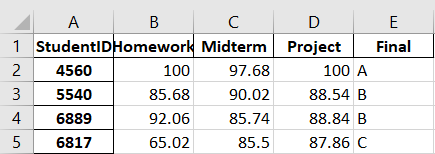If we wanted to write a single DataFrame to a single sheet with default formatting then we are done. However, if we want to write multiple sheets and/or multiple DataFrames, then we will need to create an `ExcelWriter` object.

Caution: If the `sheet_name` option of the `to_excel` method is specified in this simple use case, the resulting file will contain only the DataFrame on a single sheet of the given name. Further calls of `to_excel` with different sheet names will only overwrite the first sheet instead of adding additional sheets. An `ExcelWriter` object will need to be used to add multiple sheets.

## Opening an Excel file with Pandas ExcelWriter

To perform more complex DataFrame writes, we will need to create an `ExcelWriter` object. The `ExcelWriter` object included in the Pandas module behaves almost exactly like the vanilla Python `open` object that we used in our tutorial on Python IO. The object opens the file, handles write operations, and can be used within a `with` block.

We can open an `ExcelWriter` object within a `with` block and assign it to the variable `ew` as follows:

``````with pd.ExcelWriter(outputFile) as ew:
# Write operations...``````

And continuing the similarity to the `open` object, we can use the `to_excel` method similar to the `open` object’s `read` method. Instead of including the file pathname in the `to_excel` call, we will use the `ExcelWriter` object `ew` instead:

``````with pd.ExcelWriter(outputFile) as ew:

which results in the same Excel file we produced in the previous section.

Want to do more with Python?

Yes, show me more free Python tips

The DataFrame we created was saved under a default Excel sheet entitled `Sheet1`. If we want to change the name of the created sheet, we can use `sheet_name` option of `to_excel`:

``````with pd.ExcelWriter(outputFile) as ew:

Now that we have created the `ExcelWriter` object, we can save multiple DataFrames to multiple sheets in the Excel file:

``````with pd.ExcelWriter(outputFile) as ew:

Now our Excel file has two sheets with copies of the grades data from our Pandas dataframe.

Caution: When the `ExcelWriter` object is executed, any existing file with the same name as the output file will be overwritten. Even if the `to_excel` function writes to an unused sheet name, the file will still be overwritten in its entirety. The `ExcelWriter` class has a `mode` option that is intended to allow for appending to an existing Excel file, however this option has not been implemented on the native engine as of the writing of this tutorial.

## Writing to an Excel file with Pandas to_excel method

As we have explored in the previous section, the `to_excel` method is the primary tool for writing a Pandas DataFrame to an Excel file. The `to_excel` method has several options that give you more flexibility with how your data is saved.

### Positioning Data with `startrow` and `startcol`

Suppose we wanted to insert the grades data into the spreadsheet file in a position somewhere other than the top-left corner. We can shift where the `to_excel` method writes the data by using `startrow` to set the cell after which the first row will be printed, and `startcol` to set which cell after which the first column will be printed.

``````with pd.ExcelWriter(outputFile) as ew:

which results in the following file: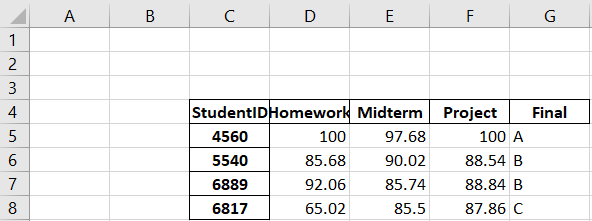Note that these inputs indicate the cell after the one given. For example, this means that if `startrow=2` then the first row of data will be printed on row 3. The header and index are considered rows and columns in this calculation.

### Formatting Float Data with `float_format`

It can be difficult to predict the number of decimals produced when writing float data created by Python. Calculations made within the DataFrames may print more decimals of precision than can be reasonably expected. To control how Python writes float data to the Excel file we can use the `float_format` option of `to_excel`.

`float_format` takes a string containing a float output format statement. See our Python String Operations and Output Formatting tutorial for more information on how to create these float format statements.

``````with pd.ExcelWriter(outputFile) as ew:
grades.to_excel(ew, float_format="%.1f") # Output only 1 decimal``````

We can now see that our output Excel file data contains only one decimal of precision: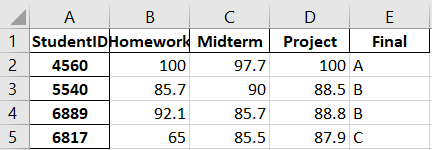Want to do more with Python?

Yes, show me more free Python tips

### Controlling the Header and Index Output

On the previous examples, the header and index of the DataFrame were printed to the Excel file along with the data. If we wish to change either of these features, we can use the `to_excel` method’s options `header` and `index`.

The `header` option can take two types of input: a Boolean to toggle printing the DataFrame’s header, or a sequence of strings to print instead of the DataFrame’s column names.

If we want to turn off the header, we can set the `header` option to false:

``````with pd.ExcelWriter(outputFile) as ew: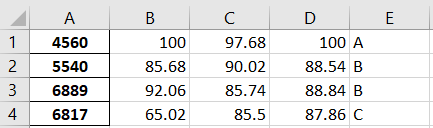We can use a new header alias by creating a list of strings and assigning it to the `header` option:

``````alias = ["A", "B", "C", "D"]
with pd.ExcelWriter(outputFile) as ew: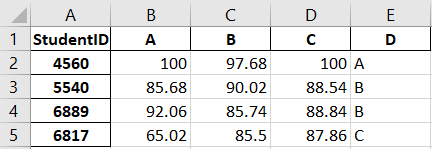Notice that the label for the index has not been changed. If we want to change the index header label, we will need to provide another alias to the `index_label` option:

``````index_alias = "I"
alias = ["A", "B", "C", "D"]
with pd.ExcelWriter(outputFile) as ew: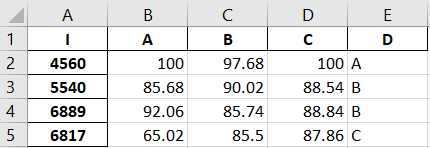We can also remove the index entirely using the `index` option:

``````with pd.ExcelWriter(outputFile) as ew: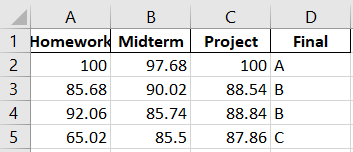Unlike the `header` option, the `index` option does not take a string of aliases. Aliasing indices currently cannot be performed with the `to_excel` method. Any changes to the indices will have to be done within the DataFrame itself prior to exporting.

Did you find this free tutorial helpful? Share this article with your friends, classmates, and coworkers on Facebook and Twitter! When you spread the word on social media, you’re helping us grow so we can continue to provide free tutorials like this one for years to come. Once you’re done sharing our tutorial, please subscribe to our Python training list using the form below:

Want to do more with Python?